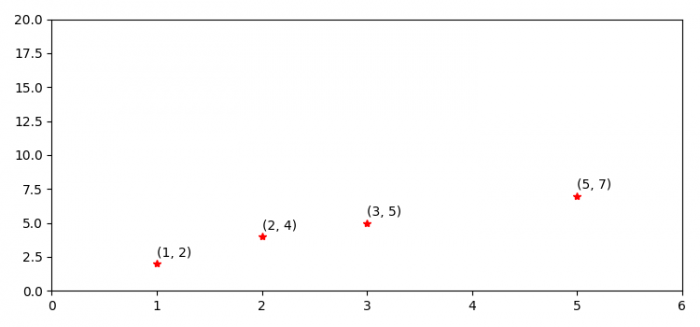# Showing points coordinates in a plot in Python using Matplotlib

PythonServer Side ProgrammingProgramming

#### Beyond Basic Programming - Intermediate Python

Most Popular

36 Lectures 3 hours

#### Practical Machine Learning using Python

Best Seller

91 Lectures 23.5 hours

#### Practical Data Science using Python

22 Lectures 6 hours

To show points coordinate in a plot in Python, we can take the following steps −

• Set the figure size and adjust the padding between and around the subplots.
• Create lists of x and y data points.
• Plot x and y data points with red color and starred marker
• Set some axis properties.
• Iterate x and y to show the coordinates on the plot.

## Example

import matplotlib.pyplot as plt

plt.rcParams["figure.figsize"] = [7.50, 3.50]
plt.rcParams["figure.autolayout"] = True

x = [3, 1, 2, 5]
y = [5, 2, 4, 7]

plt.plot(x, y, 'r*')
plt.axis([0, 6, 0, 20])

for i, j in zip(x, y):
plt.text(i, j+0.5, '({}, {})'.format(i, j))

plt.show()

## Output